# JavaScript 高性能数组去重

```// distinct.js

let arr1 = Array.from(new Array(100000), (x, index)=>{
return index
})

let arr2 = Array.from(new Array(50000), (x, index)=>{
return index+index
})

let start = new Date().getTime()
console.log('开始数组去重')

function distinct(a, b) {
// 数组去重
}

console.log('去重后的长度', distinct(arr1, arr2).length)

let end = new Date().getTime()
console.log('耗时', end - start)```

```function distinct(a, b) {
let arr = a.concat(b);
return arr.filter((item, index)=> {
return arr.indexOf(item) === index
})
}```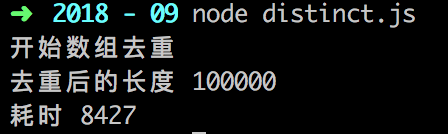```function distinct(a, b) {
let arr = a.concat(b);
for (let i=0, len=arr.length; i<len; i++) {
for (let j=i+1; j<len; j++) {
if (arr[i] == arr[j]) {
arr.splice(j, 1);
// splice 会改变数组长度，所以要将数组长度 len 和下标 j 减一
len--;
j--;
}
}
}
return arr
}```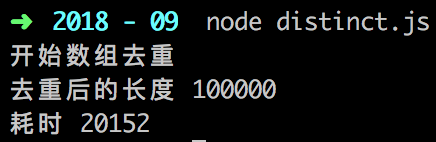```function distinct(a, b) {
let arr = a.concat(b)
let result = []
for (let i of arr) {
!result.includes(i) && result.push(i)
}
return result
}```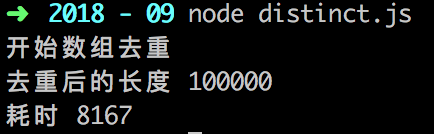```function distinct(a, b) {
let arr = a.concat(b)
arr = arr.sort()
let result = [arr]

for (let i=1, len=arr.length; i<len; i++) {
arr[i] !== arr[i-1] && result.push(arr[i])
}
return result
}```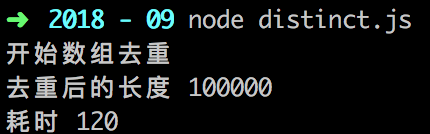ES6 新增了 Set 这一数据结构，类似于数组，但 Set 的成员具有唯一性

```function distinct(a, b) {
return Array.from(new Set([...a, ...b]))
}```

那使用 Set 又需要多久时间来处理 15W 的数据呢？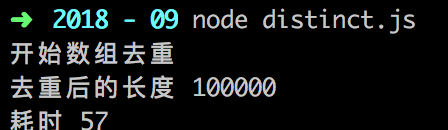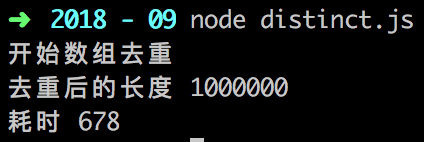```function distinct(a, b) {
let arr = a.concat(b)
let result = []
let obj = {}

for (let i of arr) {
if (!obj[i]) {
result.push(i)
obj[i] = 1
}
}

return result
}```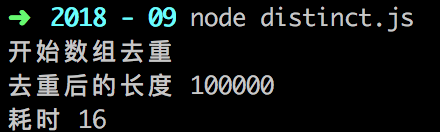15W 的数据居然只要 16ms ？？？ 比 Set() 还快？？？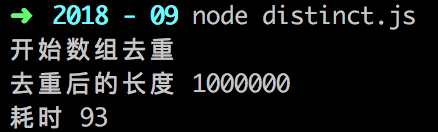emmmmmmm.... 惹不起惹不起...120 字之内